# Tricomi problem

The problem related to the existence of solutions for differential equations of mixed elliptic-hyperbolic type with two independent variables in an open domainof special shape. The domaincan be decomposed into the union of two subdomainsand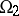by a smooth simple curve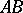whose end pointsandare different points of. The equation is elliptic in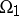, hyperbolic in, and degenerates to parabolic on the curve. The boundary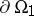is the union of the curveand a smooth simple curve, whileis the union of characteristics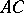and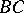and the curve. The desired solution must take prescribed data onand on only one of the characteristicsand(see Mixed-type differential equation).

The Tricomi problem for the Tricomi equation(1)

was first posed and studied by F. Tricomi , . The domainis bounded by a smooth curve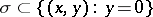with end points,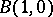and characteristicsand: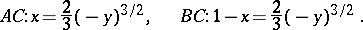Under specified restrictions on the smoothness of the given functions and the behaviour of the derivativeof the solutionat the pointsand, the Tricomi problem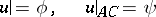(2)

for equation (1) reduces to finding the solution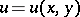of equation (1) that is regular in the domain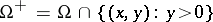and that satisfies the boundary conditions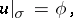(3)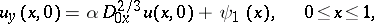where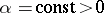,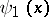is uniquely determined by,is the fractional differentiation operator of order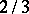(in the sense of Riemann–Liouville):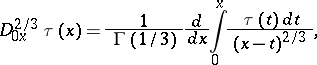and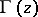is the Euler gamma-function.

The solution of the problem (1), (3) reduces in turn to finding the function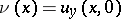from some singular integral equation. This equation in the case whenis the curvehas the formwhereis expressed explicitly in terms ofand, and the integral is understood in the sense of the Cauchy principal value (see ).

In the proof of the uniqueness and existence of the solution of the Tricomi problem, in addition to the Bitsadze extremum principle (see Mixed-type differential equation) and the method of integral equations, the so-calledmethod is used, the essence of which is to construct for a given second-order differential operator(for example,) with domain of definition, a first-order differential operator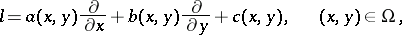with the property thatwhere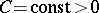andis a certain norm (see ).

The Tricomi problem has been generalized both to the case of mixed-type differential equations with curves of parabolic degeneracy (see ) and to the case of equations of mixed hyperbolic-parabolic type (see ).